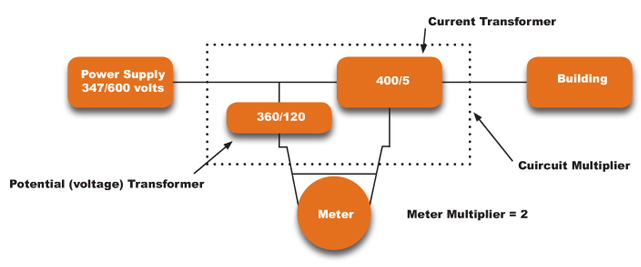COVID-19 Update: When delivering all energy efficiency programs, your health and safety is our priority. Learn more.

# Understanding the Multiplier

The actual voltage/current used is often too large to be registered by your meter.

The meter’s registering capacity may only represent a small percentage of your actual load. For example, although the actual distance between two cities may be 400 kilometers, it would be represented by only 20 millimetres on a map. The meter multiplier is similar to a map scale in that it relates to the meter's scaled down reading of the actual consumption. A metering installation has both an internal and external multiplier.  The product of these two factors provides the billing multiplier.

Current transformers and voltage or potential transformers are used to reduce the current and voltage before it enters the meter. Transformer ratios are then used to determine the external multiplier.

Therefore, on many demand meters, the metering system multiplier to be used on the registered energy usage and demand is a combination of the internal and external multipliers.

Example:

Assume that your operation requires 347/600 volts and 400 amps. This voltage and current must be reduced or stepped down by transformers before entering the meter. The amount by which the voltage and current are reduced is known as the circuit multiplier.Potential transformers (PT.Mult.) = 360 ÷ 120 = 3

Current transformers (CT. Mult)   = 400 ÷ 5 = 80

Circuit multiplier = (PT.Mult.) x (CT.Mult.) – 3 x 80 = 240

The circuit multiplier (external) is not indicated on your meter.

Billing multiplier – (circuit multiplier) x (meter multiplier) = 240 x 2 = 480.

This billing multiplier is indicated on your account invoice.

The meter multiplier (internal) shown on the face of the meter is a result of the mechanical workings of the meter.

Used for transforming primary voltage into reduced secondary voltage in metering.
Used for transforming primary current into reduced secondary current in metering.
The number by which a demand reading is multiplied, to obtain actual usage data.
For some meters, the actual amount of energy displayed is too large to be registered and the meter shows a fraction of the actual use. A multiplier is then applied to the difference between your present and previous readings to determine your actual energy use. In some cases, external multipliers may be applied (CT’s and PT’s).